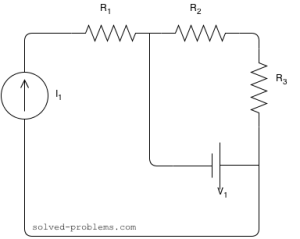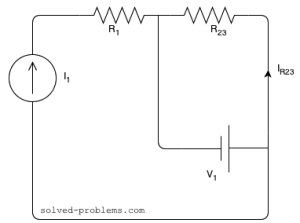Find the voltage across the current source and the current passing through the voltage source.Assume that,,,,,,

Solutionis in series with the current source; therefore, the same current passing through it as the current source:and the voltage acrosscan be found by Ohm’s law:To find the voltage across the current source, KVL can be applied around the left hand side loop:The direction does not matter and would not change the result..andare also in series and their equivalent isis parallel with the voltage source. This means that its voltage is equal to the voltage of the voltage source.Now, using the Ohm’s law, the current passing throughcan be calculated:To find the current of the voltage source, we can apply KCL at one of the nodes:Now, you tell me below what the power of each sources are?

Hi! Yaz is here. I am passionate about learning and teaching. I try to explain every detail simultaneously with examples to ensure that students will remember them later too.

## Join the Conversation

1.2.3.4.5.1. plz solve exercise problems on Nortons theorem from BE 3 Sem na books

1. Have you checked out ?
And let me know which problem you would like me to solve.

2.ramasubramanian says:

i will need some kvl&kcl simple problem

3.selvasubramaniyan says:

super

4. This site is really for us student….will like to keep in touch